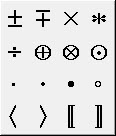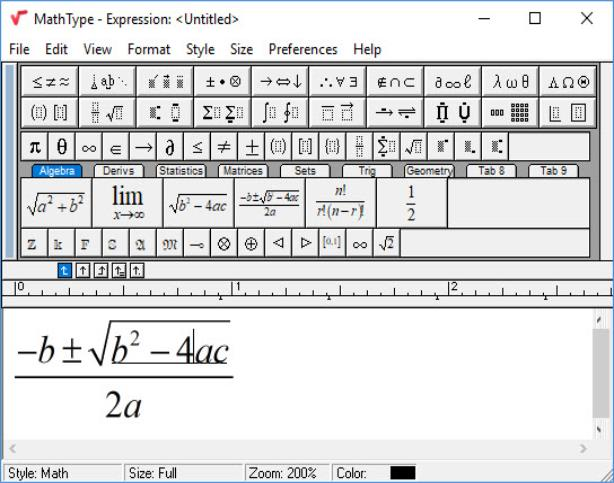### MathType™

Note: As of October 2018 the current version of MathType is 7.4.

MathType, produced by Design Science, Inc., is a relatively low-cost add-on for Word for Windows which enables mathematical formulae to be inserted into Word documents. These documents containing MathType equations can be opened in DBT and translated into mathematical braille.

Modern day mathematical editors make extensive use of Unicode for mathematical signs. Unfortunately, visually, many signs appear alike, or nearly so. Also, we have become used to using the PC keyboard for inputting all arithmetical or mathematical characters. Instead, MathType provides menus for selecting the correct signs and symbols.For example, two times two equals four, is often keyed in as: "2x2=4" using the "x" character.

Using correct mathematical input it should appear as "2×2=4".

In the second case you will see that the x is a proper multiplication sign, raised above the baseline.

Fortunately, MathType features allow you to easily select the correct symbols, only provided that you know what you require. (We recommend that the preparation of mathematical material should be done by those with a good knowledge and understanding of mathematics.)

This is very simple. Let us say we want to insert the following equation into Word:Where, if we wished to see this equation in TeX, it would appear as follows:

$\frac{-b\pm \sqrt{{{b}^{2}}-4ac}}{2a}$

Or, for LaTex, it would be slightly different (but still as complex):

$\frac{{ - b \pm \sqrt {{b^2} - 4ac} }}{{2a}}$

In Word, you just click on MathType and then "Inline Equation" (Word 2003) or "Inline" (Word 2007/2010). You will see the following dialog into which you enter your equation. When you have completed the equation, close the MathType window, and your equation will appear in the document.

It is beyond the scope of this topic to fully explain how MathType is used, but the product itself contains excellent instructions.